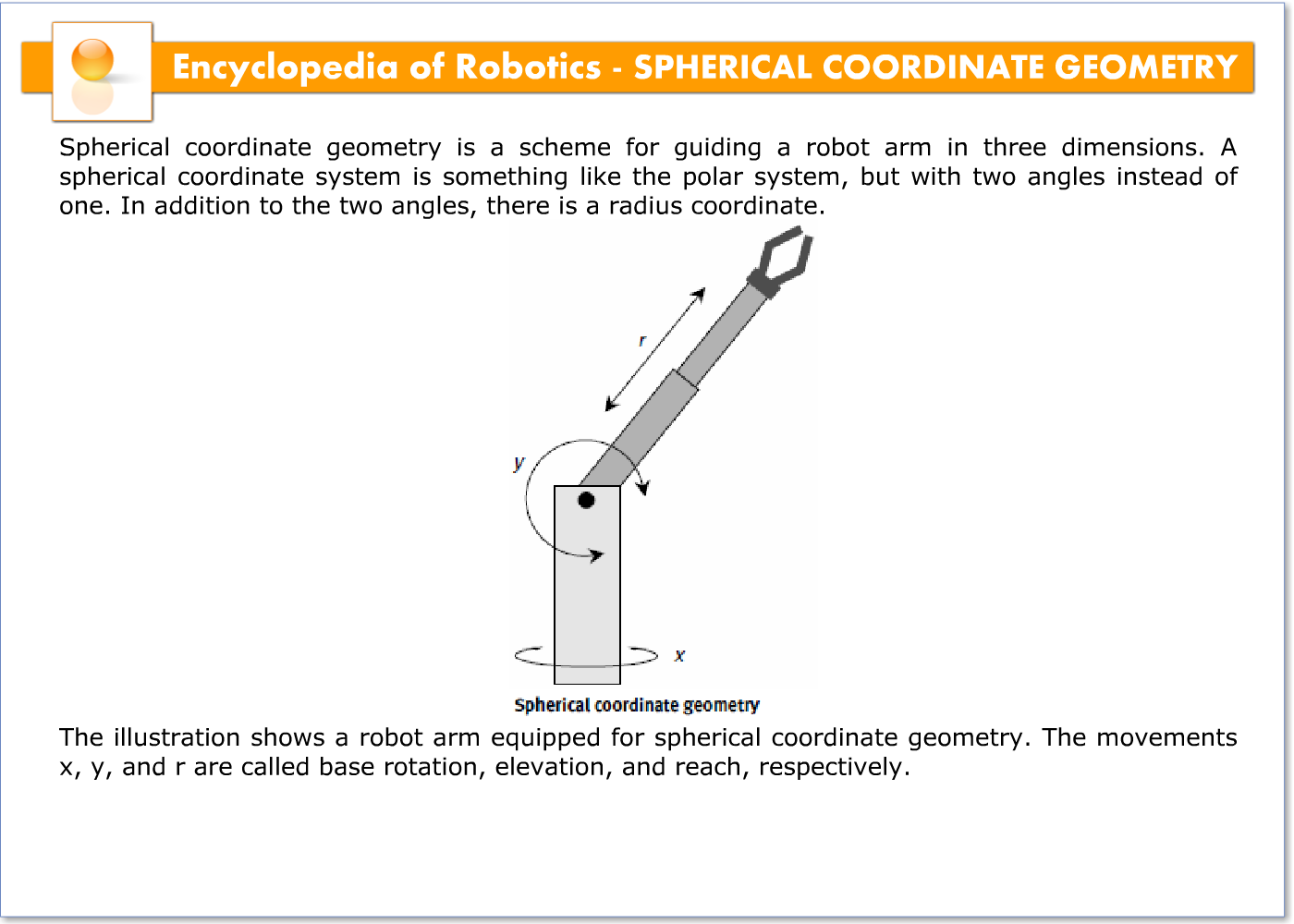# SPHERICAL COORDINATE GEOMETRY – 21127

Spherical coordinate geometry is a scheme for guiding a robot arm in three dimensions. A spherical coordinate system is something like the polar system, but with two angles instead of one. In addition to the two angles, there is a radius coordinate.One angle, call it x, is measured counterclockwise from the reference axis. The value of x can range from 0° to 360°. You might think of x as similar to the azimuth bearing used by astronomers and navigators, except that it is measured counterclockwise rather than clockwise. As a ray rotates around a full circle through all possible values of x, it defines a reference plane.

The second angle, call it y, is measured either upward or downward from the reference plane. The value of y will ideally range from 90° (straight down) to +90° (straight up). Structural limitations of the robot arm might limit the lower end of this range to something like 70°. You might think of y as the elevation above or below the horizon.

The radius, denoted r, is a non-negative real number (zero or greater). It can be specified in units such as centimeters, millimeters, or inches.

The illustration shows a robot arm equipped for spherical coordinate geometry. The movements x, y, and r are called base rotation, elevation, and reach, respectively.